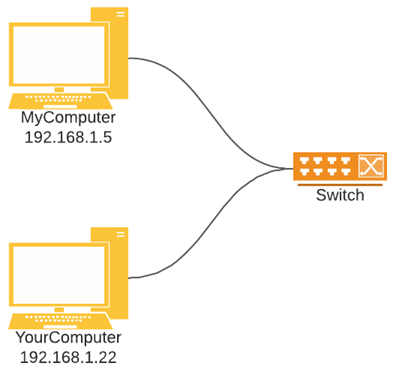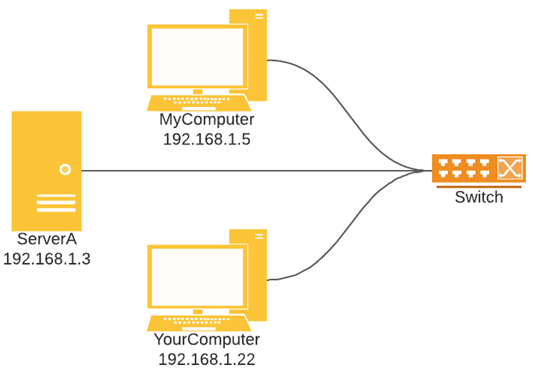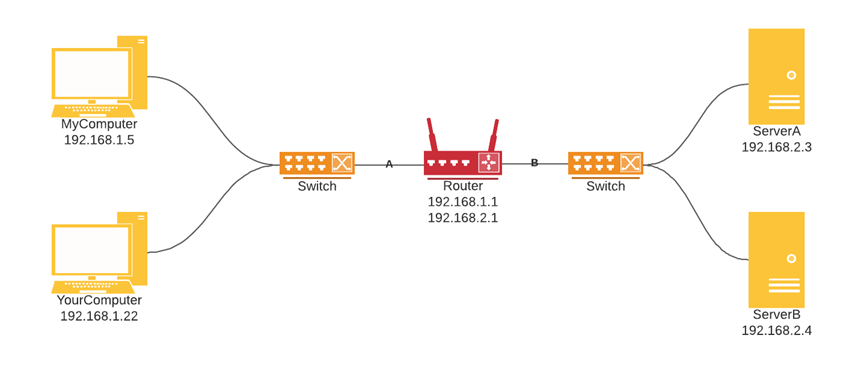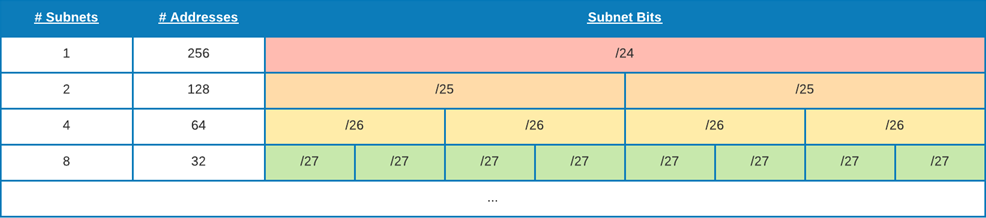# What is subnetting?

Matthew Fisher - August 22, 2021Learn to master subnets: IP addresses, networks, subnets, and subnet calculations.

Modern networks are built to be subdivided into sub-networks known as subnets. In this article, describe the concepts and calculations involved in building and managing subnets.

As a companion to this detailed article, download my free PDF subnet chart, which is an excellent reference for subnets.

## IP Networks

An IP address is the network address of a device, specified in a dotted decimal notation (www.xxx.yyy.zzz) or a as a binary number. A network device can be a server, a piece of network equipment, a client computer, a mobile phone, or something else. Any device capable of communicating on an IP network needs an IP address to participate on the network.

There are both public and private IP addresses. Public IP addresses are visible on the public Internet. Do you know your public IP address?

### Networks

Let's build up a concept of a network next. Consider the following network diagram:Suppose you have two computers, MyComputer and YourComputer. The computers need to be able to communicate with each other, so they are given IP addresses (MyComputer is given 192.168.1.5 and YourComputer is given 192.168.1.22). The two computers have unique IP addresses on the network, but no way of sending and receiving data.

A switch is a network device that connects devices by relaying packets they send to other devices on the network. MyComputer and YourComputer are both connected to the switch via a network cable in this example. This allows MyComputer to send data to YourComputer by sending a packet across the network cable. The switch will intercept the packet and forward it to other devices connected to it.

Now suppose this network needs a server to host a website that MyComputer and YourComputer can access:Following the same network principle as before, MyComputer, YourComputer, and ServerA are all connected by a network cable to a switch. Any communications between devices in this network are handled by the switch and relayed to the appropriate host or hosts.

### Routing and Switching

Let's add one more detail to this - a requirement to separate workstation traffic from server traffic:Switches relay data packets to all directly connected devices. To allow for the separation of communications between devices, a router is used. A router is a network device that selects the network path for communications to follow based on a defined set of rules. In the diagram above, the router would be responsible for routing data to path A or path B based on a set of rules. Examples of rules the router could follow are:

• Allow web traffic from YourComputer to ServerB.
• Deny any SSH traffic from path A to path B.

The general rules to take away from routing and switching are the following:

• A switch allows network communications to occur between connected hosts.
• A router allows network communications to occur across segments of a network, subject to routing rules.

### Subnets

On each side of the router in this network is a subdivision of the network, or a subnet. All of the workstations can communicate directly with each other, all of the servers can communicate directly with each other, but traffic across subnets must traverse the router and be subjected to the router's ruleset. We will describe the mechanics of subdividing a network into subnets below.

From this perspective, a network is a system of interconnected devices, each of which having an IP address, whose communications are mediated by network equipment such as routers and switches.

## Network Subdivision

### Subnets

A subnet is a logical subdivision of a larger network into non-overlapping IP address ranges. It could be as small as a single host or as large as an entire internetwork. It is desirable to subdivide a network for many reasons. The primary reasons for subdividing a network are:

• To improve routing efficiency by reducing the number of devices that communicate directly with each other.
• To create isolation between logically related devices, such as workstations and servers.

Every IP address on a network has at least two components that define it: the IP address and a subnet mask. A subnet mask is a 32-bit binary number that specifies how a network should be subdivided into subnets.

Let's look at an example:

The network address of a network is `10.0.1.0/24`.

This network has a network address (the first address in the network) of 10.0.1.0. The "/24" is a part of CIDR notation that indicates 24 bits are to be used for the subnet mask. Given the subnet mask of 24, we can conclude that the first 24 out of 32 bits in the address are used to distinguish different subnets, while the remaining 8 bits are used to distinguish hosts within a subnet. We use bits in subnetting, since IP addresses and subnet masks can be represented in binary notation:

``````10.0.1.0
00001010 00000000 00000001 00000000
``````

``````/24
255.255.255.0
11111111 11111111 11111111 00000000
``````

When subdividing networks, each additional bit used for the subnet mask results in twice as many subnets, each half the size. A /24 (read: "slash twenty four") subnet can be subdivided by adding one bit to the subnet mask, making it a /25 subnet. When doing so, the 256 addresses in a single subnet become 128 addresses in two subnets. Subdividing a /25 into a /26 likewise doubles the number of subnets and halves the number of addresses in each subnet. The following table illustrates this subdivision process:The procedure for subdividing a network into subnets is as follows:

1. Increase the number of subnet bits by 1.
2. Each time you add 1 subnet bit, the resulting address space will have twice as many subnets with half as many addresses each.

## Subnet Calculations

Subnet calculations are possible if we convert IP addresses from dotted decimal notation to binary numbers. Since an IP address consists of four octets ranging from 0 to 255, separated by a period, the same IP address can be represented by converting each octet into an 8-digit binary number and removing the periods between octets.

In this example, we convert the IP address `10.0.1.0/24` from dotted decimal notation to binary:

``````10.0.1.0
00001010 00000000 00000001 00000000
``````

Next, we convert the subnet mask into binary by listing 24 ones followed by 8 zeroes:

``````/24
11111111 11111111 11111111 00000000
``````

Now if we put the network address and subnet mask together, we can see that all the IP address bits that correspond to a one in the subnet mask are used to define the subnet. The remaining bits correspond to a specific IP address within the subnet:

``````00001010 00000000 00000001 00000000
11111111 11111111 11111111 00000000
``````

This entire process is made easier by using an online subnet calculator, but let's take these calculations further. Enter a CIDR address or IP address below to find the details of the network.

### Online Subnet Calculator

Look up details of IPv4 subnets and CIDR blocks.

### What is the Network Address for a Subnet?

To determine the network address (network prefix) for a subnet, perform a bitwise logical AND between an IP address in the subnet and the subnet mask, both represented in binary. Follow these steps to find network addresses:

1. Convert the IP address to binary.
2. Obtain the subnet mask for the network in binary representation.
3. Perform a bitwise logical AND on the IP address and subnet mask.
4. Convert the resulting address back to dotted decimal notation. This is the network address.

#### Example

Suppose we want to find the network address for `10.0.1.15` on a network using 24 bits for its subnet mask. The steps are shown below.

``````IP address:
10.0.1.15/24

1. Convert the IP address to binary:
00001010 00000000 00000001 00001111

2. Obtain the subnet mask in binary representation:
11111111 11111111 11111111 00000000

3. Perform a bitwise logical AND:
00001010 00000000 00000001 00001111
11111111 11111111 11111111 00000000
-----------------------------------
00001010 00000000 00000001 00000000

4. Convert to dotted decimal notation:
10.0.1.0
``````

1. Convert the IP address to binary.
2. Obtain the subnet mask for the network in binary representation.
3. Set all bits to 1 in the host portion of the IP address (where the corresponding subnet bit is a 0).

#### Example

Suppose we want to find the broadcast address for `10.0.1.15` on a network using 24 bits for its subnet mask. The steps are shown below.

``````IP address:
10.0.1.15/24

1. Convert the IP address to binary:
00001010 00000000 00000001 00001111

2. Obtain the subnet mask in binary representation:
11111111 11111111 11111111 00000000

3. Set all bits to 1 in the host portion of the IP address:
00001010 00000000 00000001 00001111
11111111 11111111 11111111 00000000
-----------------------------------
00001010 00000000 00000001 11111111

4. Convert to dotted decimal notation:
10.0.1.255
``````

Subscribe for more articles like this.